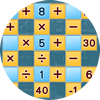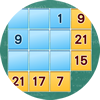Novel Games - Mastering All the Games in Human History
search Play Games Forums License Our Games
Name
0 / 2490
0
99

Settings Log Out

Garam

Game Description

This is a more complicated and harder version of Fubuki and Math Square. The goal is the same as that of Math Square, you put in numbers to make all the formulas correct. What is different in this version is that the formulas are arranged in a series of connected rings, so that the numbers you use in each of the formulas are interrelated. You can put numbers from 0 to 9, and can use a number multiple times or not at all. The formulas are shorter, there is only one operation (addition, subtraction, multiplication, or division) and only three numbers in each forumla. You need to put in the answers as well and the answers can have two digits. This game is harder than both Fubuki and Math Square because of the complicated relationship between the numbers in different formulas. The faster you finish, the higher your rank.

Submit
Submit comment failed, please try again Submit
None Yet(Translated by #[PERSON])
(Original)
by %s
Like

None Yet
by %s

You may also like:Math Square
Put in numbers to make the formulas correct.
Version: 1.0.0FubukiTime Difference